# Scatter Plot

Scatter Plot

Scatter Plots are described as the most useful invention in statistical graphs. Originally, the scatter plot was presented by an English Scientist, John Frederick W. Herschel, in the year 1833. Herschel used it in the study of the orbit of the double stars. He plotted the positional angle of the double star in relation to the year of measurement. The scatter plot was used to understand the fundamental relationship between the two measurements.Even though bar charts and line plots are frequently used, the scatter plot still dominates the scientific and business world. It is very easy for people to look at points on a scale and understand their relationship.

Let us explore this topic to understand more about scatter plots.

## Lesson Plan

 1 What Is a Scatter Plot? 2 Thinking Out of the Box! 3 Challenging Question 4 Solved Examples on Scatter Plot 5 Interactive Questions on Scatter Plot

## What Is a Scatter Plot?

A scatter plot is a means to represent data in a graphical format.

A simple scatter plot makes use of the Coordinate axis to plot the points, based on their values.

The following scatter plot excel data for age (of the child in years) and height (of the child in feet) can be represented as a scatter plot.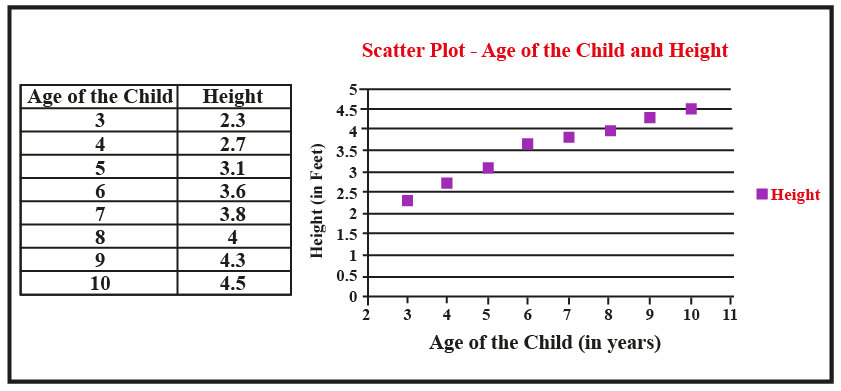A scatter plot is an easy way to visually present and understand data.

A scatter plot can also be drawn for three-dimensional data.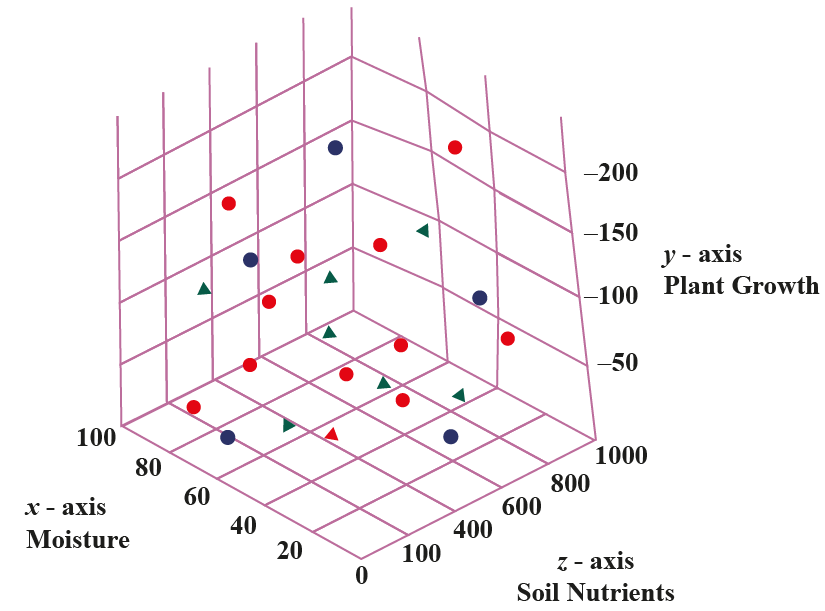Examples

(a)  Days of the week and the sales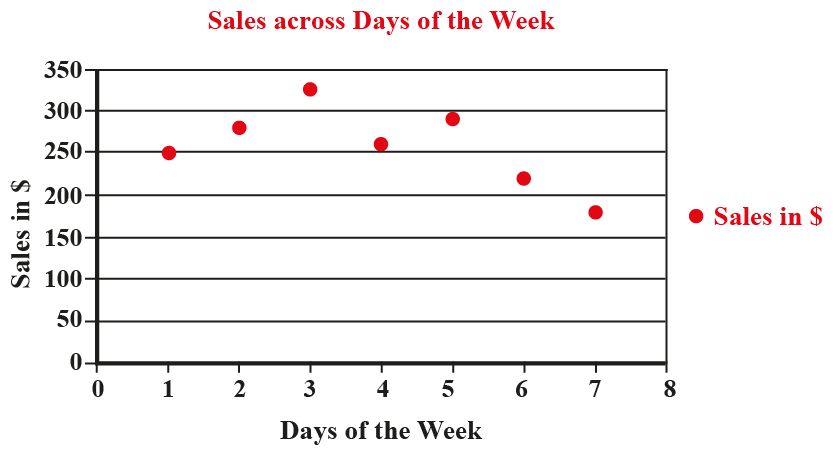(b) Age (of a person) and the percentage of savings can be shown in the below scatter plot excel.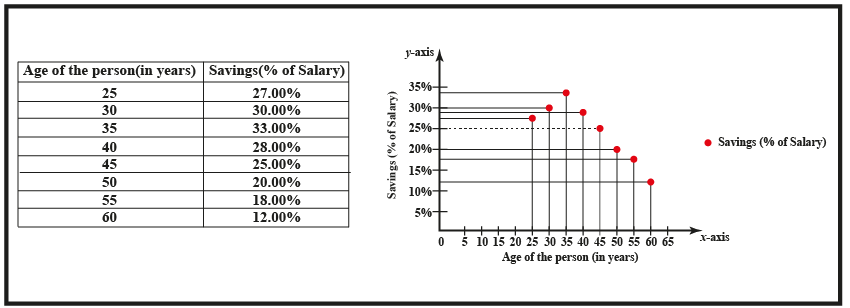Other Formats to Represent Data:

There are numerous ways to graphically represent data.

Some of the common ways are pie chart, box plot, block diagram, 3-D graph, Comparison Graphs.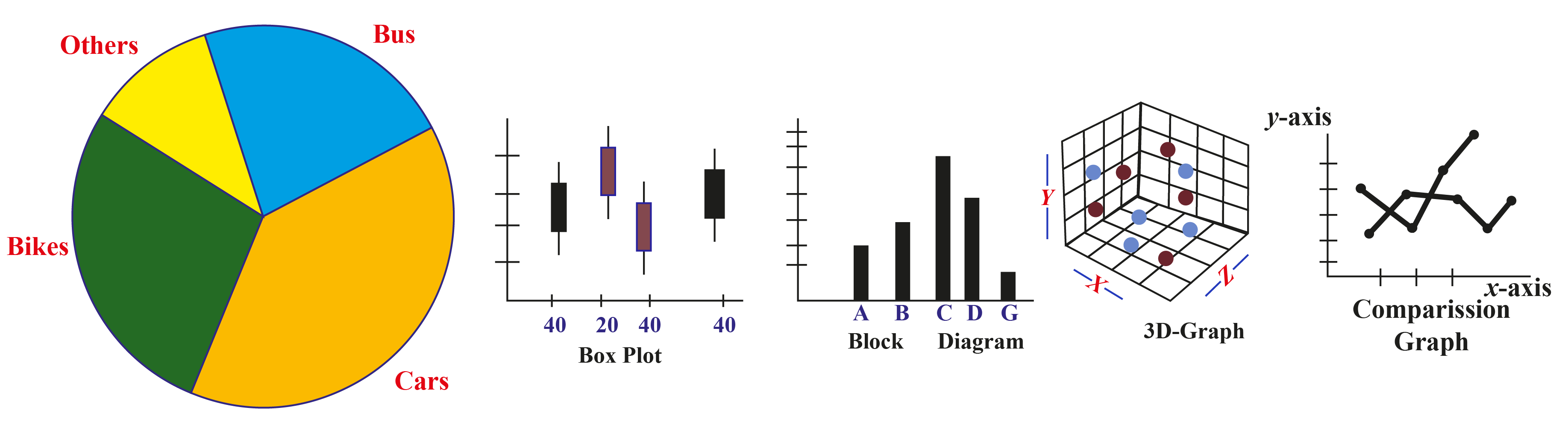## How to Construct a Scatter Plot?

There are three simple steps to plot a scatter plot.

STEP - I: Identify the x-axis and y-axis for the scatter plot.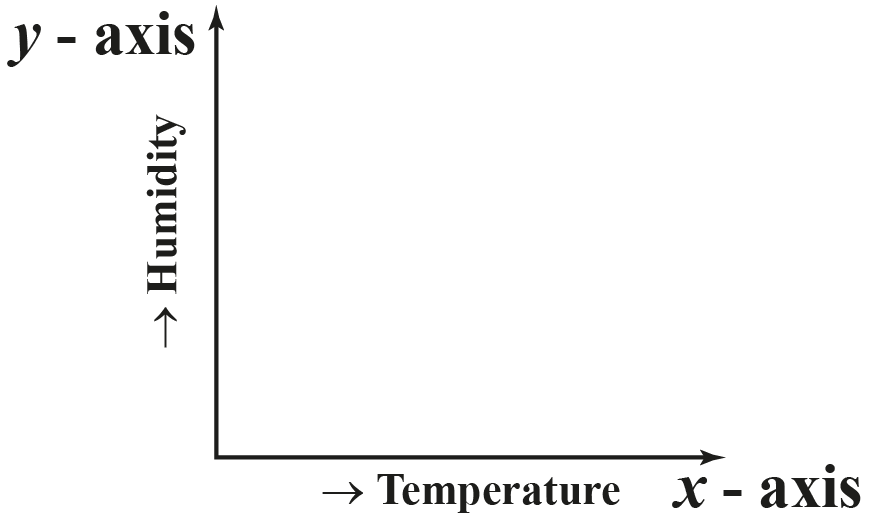STEP-II: Define the scale for each of the axes.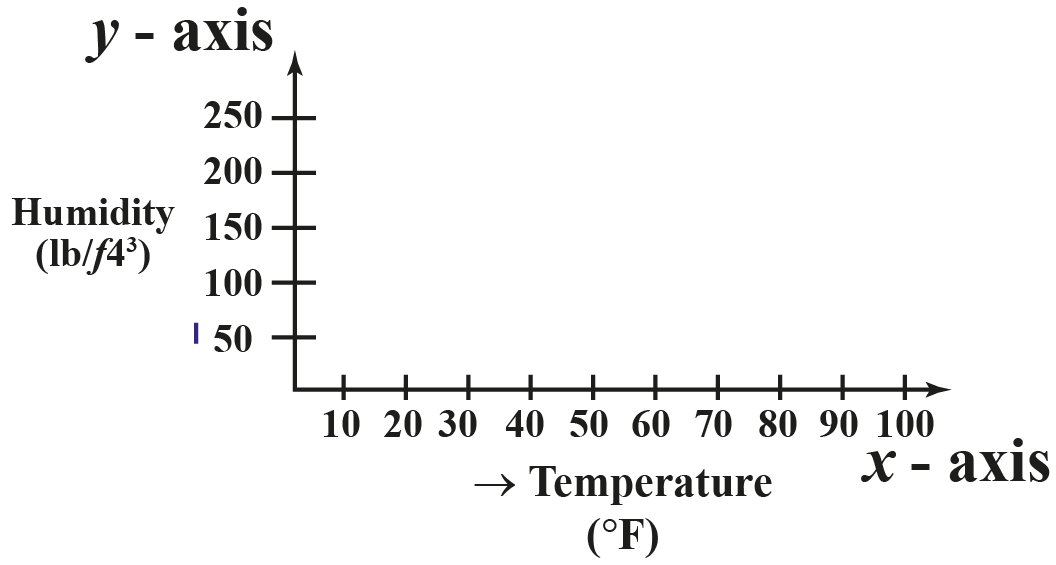STEP-III: Plot the points based on their values.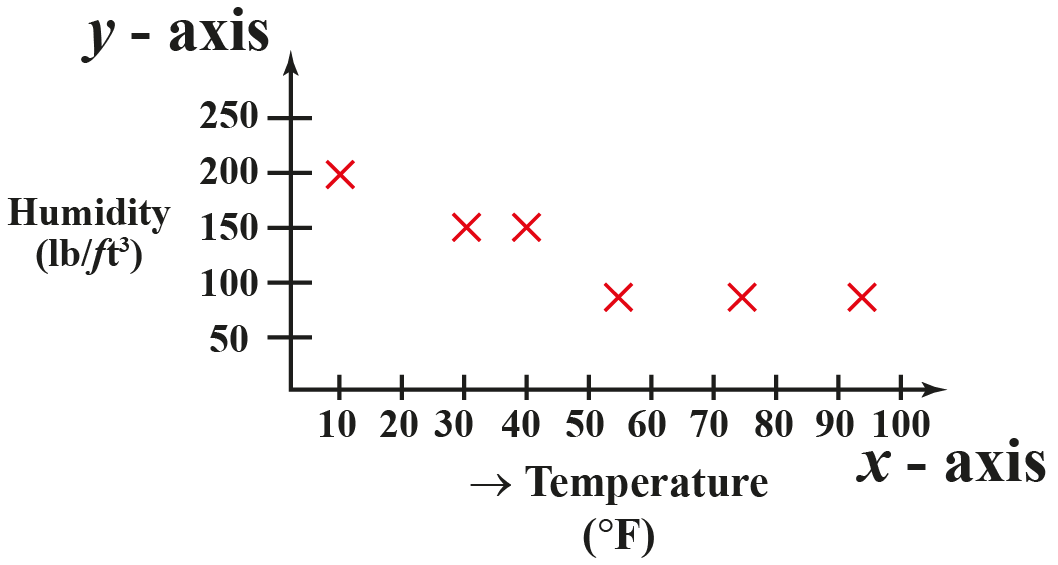Think Tank

A scatter plot represents the relationship between two variables. The values of the independent variable are marked on the x-axis and the values of the dependent variable are marked on the y-axis.

Example -1: In the scatter plot representing the days of the week and the sales on each of the days, the days of the week is the independent variable and the sales are the dependent variable.

Example-2: In the scatter plot representing the temperature and humidity of a place, temperature is the independent variable and humidity is the dependent variable.

Question: Jimmy has collected data of his employees' age and salary. Help Jimmy identify the dependent variable and the independent variable.

## Types of Scatter Plot

A scatter plot helps find the relationship between two variables

This relationship is referred to as a correlation.

Based on the correlation, scatter plots can be classified as follows.

### Positive

A scatter plot with increasing values of both the variables can be said to have a positive correlation.

The scatter plot of the time spent in studying for an examination and the marks scored can be referred to as having a positive correlation.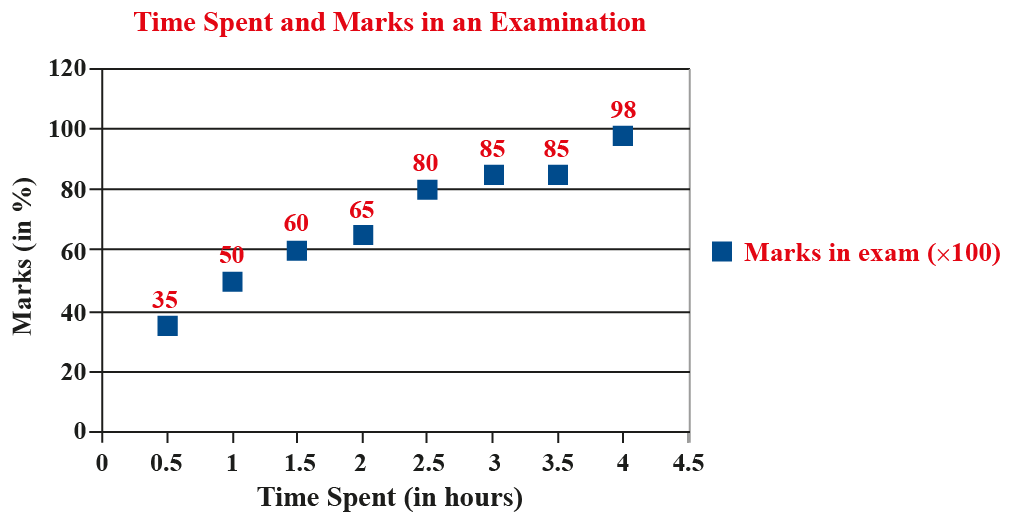### Negative

A scatter plot with an increasing value of one variable and a decreasing value for another variable can be said to have a negative correlation.

The scatter plot showing the production of crops and the price of the crop represents a negative correlation.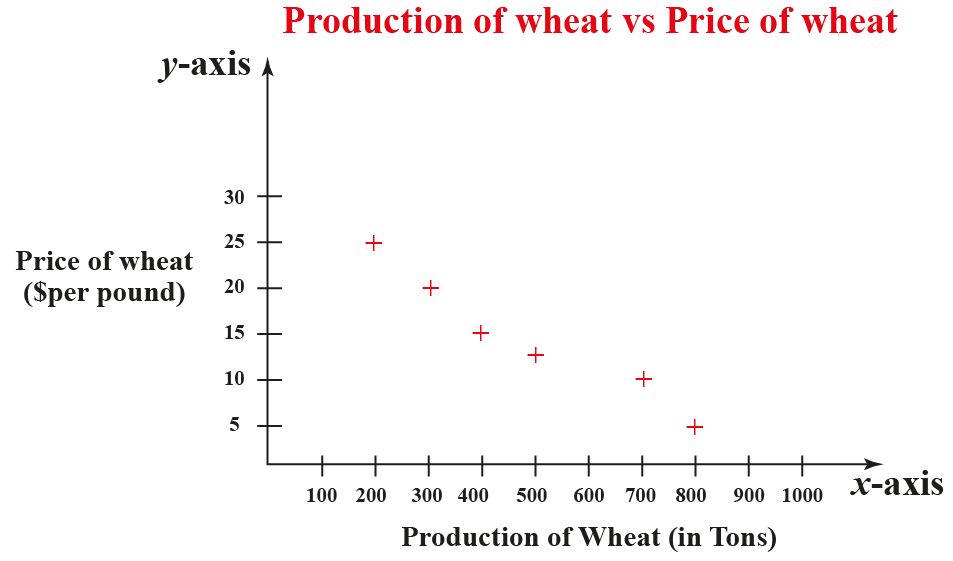### None

A scatter plot with no clear increasing or decreasing trend in the values of the variables is said to have no correlation or none

Here the points are distributed randomly across the graph.

The number of birds on a tree at different times of the day does not show any correlation.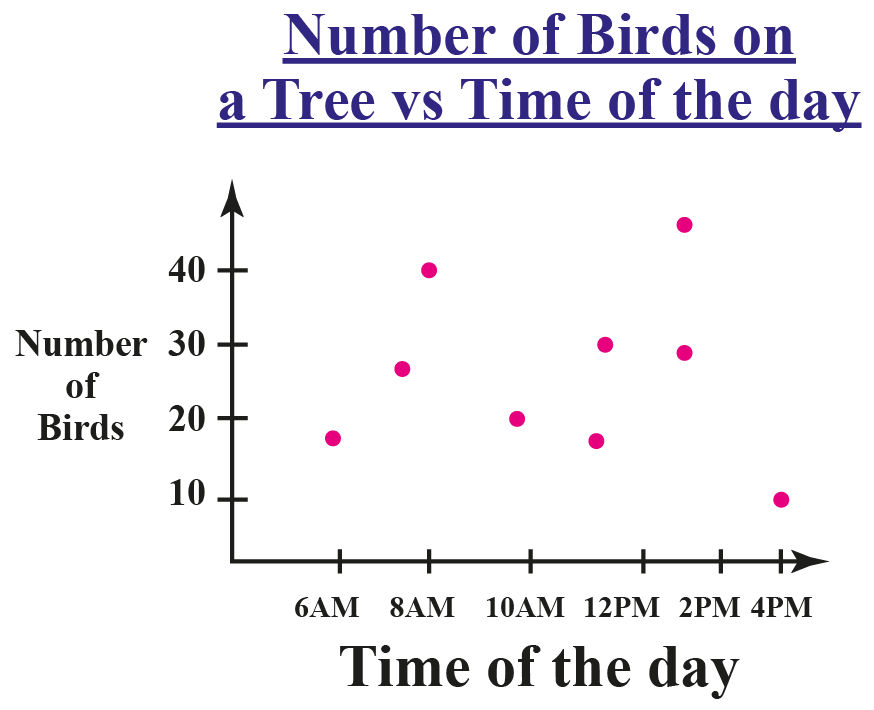Challenging Question

A chain of supermarket is aiming at analyzing the sales and profit of its ten individual stores.  The data is in the form of coordinates.

($50000, 12%), ($30000, 30%), ($42000, 23%), ($53000, 18%), ($22000, 28%), ($36000, 25%) , ($33000, 18%), ($27000, 20%), ($45000, 23%), ($,55000, 11%)

Plot the above values on a scatter plot and identify the correlation between sales and the profit percentage of the individual stores.

## How to Solve a Scatter Plot?

A scatter plot can be solved to understand the level of correlation among the set of variables.

In the below simulation, the cursor can be moved across the scale to observe the different levels of correlation based on the different arrangement of the data points.

## What Is Scatter Plot Analysis?

Analysis of a scatter plot helps us understand the following aspects about the data.

• The different levels of correlation among the data points is useful to understand the relationship within the data.
• A line of best fit can be drawn for the given data and used to further predict new data values.
• The data points lying outside the given set of data can be easily identified to find the outliers.
• The grouping of data points in a scatter plot can be identified as different clusters within the data.

## Solved Examples

 Example 1

Lawrell had visited a zoo recently and had collected the following data.

How can Lawrell use a scatter plot to represent this data?

Type of Animal Number of Animals in the Zoo
Zebra 25
Lions 5
Monkeys 50
Elephants 10
Ostriches 20

Solution

The aim is to present the above data in a scatter plot.

Step -1: Mark the points on the x-axis and write the names of the animals beside each of the markings.

Step - 2: Marks the points as 10, 20, 30, 40, 50, 60 on the y-axis to represent the number of animals.

Step - 3: Identify the animals marked on the x-axis and mark a point above it based on the number given in the table. Refer to the y-axis to measure and mark the points.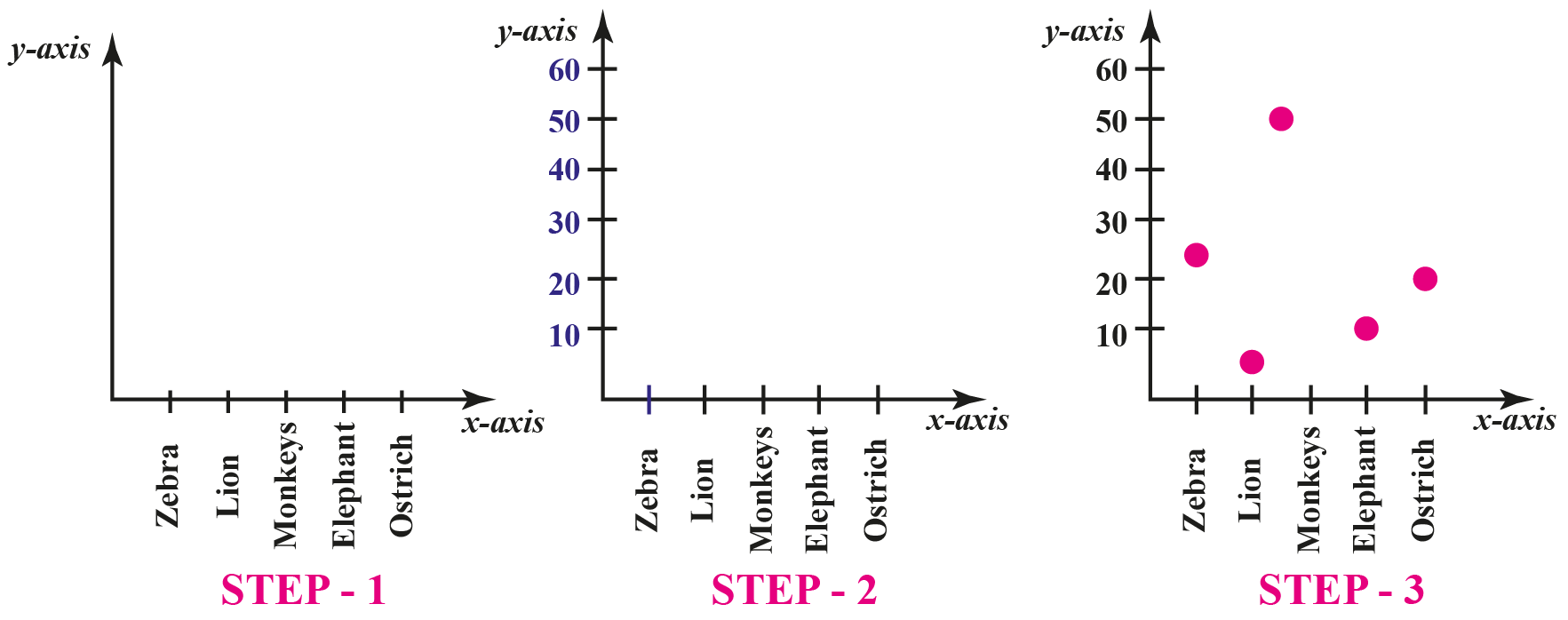$$\therefore$$ The points representing the number of animals have been plotted on the scatter plot.
 Example 2

The meteorological department has collected the following data about the temperature and humidity in their town.

Refer to the table given below and indicate the method to find the humidity at a temperature of 60 degree Fahrenheit.

Temperature (Degree Fahrenheit) Humidity(%)
45 60
62 48
77 40
97 30
118 20
122 18

Solution

The collected data of the temperature and humidity can be presented in the form of a scatter plot.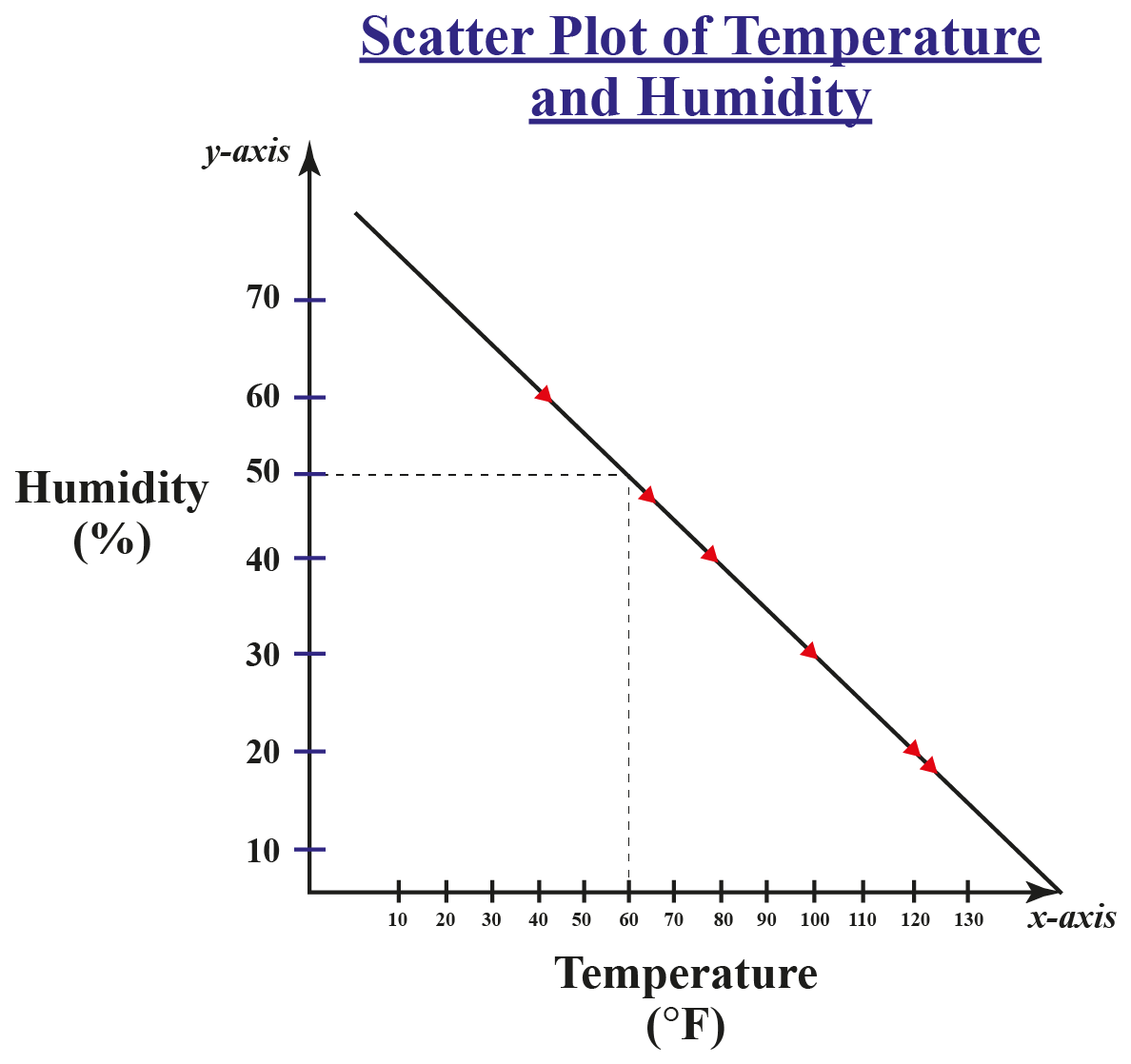Temperature is marked on the x-axis and the humidity on the y-axis.

To calculate the humidity at a temperature of 60 degree Fahrenheit, we need to first draw a line of best fit.

A line of "Best Fit" is a straight line drawn to pass through most of these data points.

Now draw a vertical line from the mark of 60 degrees Fahrenheit on the x-axis, so that it cuts the line of "Best Fit".

At the point where this line cuts the line of "Best Fit", the corresponding marking on the y-axis represents the humidity at 60 degrees Fahrenheit.

 $$\therefore$$ The humidity at a temperature of 60 degree Fahrenheit is 50%
 Example 3

In a school, a teacher has prepared a scatter plot on her computer to show the marks of 8 students and the time spent in preparation for the examination.

How can we help the teacher find the outlier?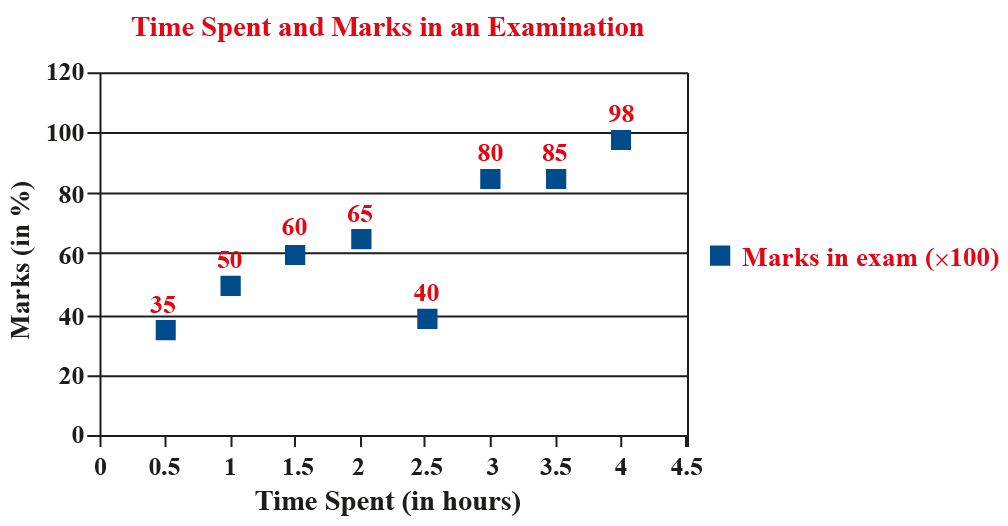Solution

The data in the scatter plot shows a positive correlation; the marks increase with an increase in time spent on preparation.

But the data point referring to the student who has to spend 2.5 hours of time for preparation and has secured 40% of marks is distinct from the correlation and can thus be identified as an outlier.

 $$\therefore$$ The data points of the student with 40% marks and time of 2.5 hours is the outlier.
 Example 4

Samuel has the following three sets of data.

Can you help Samuel decide if he can use a scatter plot for each of the sets of data?

(a)  The table below presents an analysis by a car dealer on the name of the car and their mileage.

Name of the Car Mileage (mpg)
Ford 29
Dodge 23
Chevrolet 25
Ram 20
Lincoln 23

(b)  In the table below, a farmer has listed the quantity of production of each of the food variety grown on his farm.

Food Variety Production (in tons)
Wheat 65
Rice 42
Cotton 24
Pulses 20
Turmeric 15

(b)  A supermarket has an inventory of the following items with their price per unit and the available quantity.

Name of the Item Price per unit (in \$) Quantity (Number)
Soap 3 250
Tooth Paste 2 150
Pen 2.5 300
Tumbler 5 60
Water Cans 4 110

Solution

Samuel can draw a scatter plot for each of the given data sets.

(a)  Here, a two-dimensional scatter plot can be drawn with the name of the car on the x-axis and the mileage on the y-axis.

(b)  A two-dimensional scatter plot can be drawn with the food variety presented on the x-axis and the quantity on the y-axis.

(c)  The data of the supermarket consists of three variables - Name of the Item, Price, and Quantity. Hence, a 3-dimensional scatter plot can be drawn with the Name of the Item on the x-axis, Price on the y-axis, and Quantity on the z-axis.

 $$\therefore$$ A scatter plot can be drawn for each of the given data sets.

## Interactive Questions

Here are a few activities for you to practice. Select/Type your answer and click the "Check Answer" button to see the result.

## Let's Summarize

The mini-lesson targeted the fascinating concept of scatter plot. The math journey around scatter plot starts with what a student already knows, and goes on to creatively crafting a fresh concept in the young minds. Done in a way that not only it is relatable and easy to grasp, but also will stay with them forever. Here lies the magic with Cuemath.

At Cuemath, our team of math experts is dedicated to making learning fun for our favorite readers, the students!

Through an interactive and engaging learning-teaching-learning approach, the teachers explore all angles of a topic.

Be it worksheets, online classes, doubt sessions, or any other form of relation, it’s the logical thinking and smart learning approach that we, at Cuemath, believe in.

## 1. What is interpolation and extrapolation in a scatter plot?

Interpolation or extrapolation helps in predicting the values of the new data using scatter plots.

• Interpolation helps to predict the new values for data points, within the range of the given set of data.
• Extrapolation helps to predict the new values for the data points, which are beyond the given set of data.

## 2. What is a correlation in a scatter plot?

The relationship between the different variables in data is referred to as a correlation.

Scatter plots help find the correlation within the data.

There are three types of correlation:

• Positive Correlation
• Negative Correlation
• No Correlation (None)

## 3. How can you differentiate between a positive and negative correlation on a scatter plot?

In a positive correlation, both the variables increase or decrease in a similar manner.

The line of best fit for the data points with a positive correlation would have a positive slope

Further, in a negative correlation, one variable increases, and another variable value would decrease.

The line of best fit for the data with negative correlation would have a negative slope.

More Important Topics
Numbers
Algebra
Geometry
Measurement
Money
Data
Trigonometry
Calculus